# Sparsity and interpretability?

A series of interactive visualisations of small-scale experiments to generate intuitions for the question: “Are sparse models more interpretable? If so, why?”## Assumptions and motivation

• Understanding interpretability may help AI alignment directly (e.g. recursive approaches, short/​mid-term value; see here) or indirectly by supporting other directions (mechanistic transparency, open-source game theory).

• Recent deep learning sparsification techniques retain most of the network’s performance (even when dropping 99% of the weights).

• At least small systems are easier to both analyze when sparse (e.g. information flow, case debugging, local behaviour interpretation) and explore & visualize.

• Experiments with small networks can generate or challenge general intuitions (with some caveats) and inform further technical research.

• In particular, we assume that moving towards early, simplified or naive definitions of interpretability concepts and other engagement are useful steps towards more general understanding (as one possible way).

• We want more people to engage with these questions by playing with hands-on examples and challenging & debating concrete propositions. Reach out and comment!

Many of the visualisations here are interactive. Due to technical forum limitations, the interactive versions are on an external page here and are linked to from every visualisation snapshot in this post.

# Introduction [Optional]

We want to understand under which circumstances (if any) a sparser model is more interpretable. A mathematical formulation of “transparent or interpretable” is currently not available: sparsity has been proposed as a possible candidate proxy for these properties, and so we want to investigate how well the links between sparsity and interpretability holds, and what kinds of sparsity aid in what kinds of interpretability.

(A note on the field of Interpretability: we’ve talked a bit previously about what interpretability is, as the topic is broad and the term is very underdefined. It’s important to note, when discussing whether a particular property (like sparsity) makes a model more or less interpretable, we need to specify what kind of interpretability method we’re using, as different methods will benefit from different properties of the model.)

We have several intuitions that sparsity in neural networks might imply greater interpretability, and some which point in the opposite direction:

• Debugging a system with many weights and interconnections seems more complex than to do the same for a sparser system with less parameters and connections; High connectivity is one of reasons why neural networks are not considered transparent.

• On the other hand, human-recognizable features are often complex objects—such as in an image classification task, where humans recognize faces, dog ears, and trees as opposed to simple patterns of white noise. There is hence a risk that making a system sparser could lessen its capacity to work with such high-level concepts.

• In other words, a dense system may be able to use complex but human-interpretable features, whereas a sparse system wouldn’t have the capacity to recognise these features and hence focus on simpler features which are less meaningful to humans.

• Neural networks trained against adversarial attacks produce more human recognizable features . If making the system sparser makes it more vulnerable to adversarial attacks, then it may then be less human-interpretable. However, it is unclear in what direction the causality would flow.

In this post we investigate a specific instantiation of an interpretability method which intuitively seems to benefit greatly from sparsity, and discuss ways in which we can make the most of these benefits while not changing the network to be too sparse and lose performance

# Introduction of the Experiment

To test whether sparsity is good for interpretability, the obvious thing to do is to get a sparse neural network, and see whether it’s easier to interpret. To do it we need following things: a neural network, a pruning method to produce a sparse network, and an interpretability method to apply to this sparse network.

Neural network. We will use a simple network of the following architecture trained on MNIST (2 hidden layers of size 32 with ReLU activations; in total 26432 weights in kernels):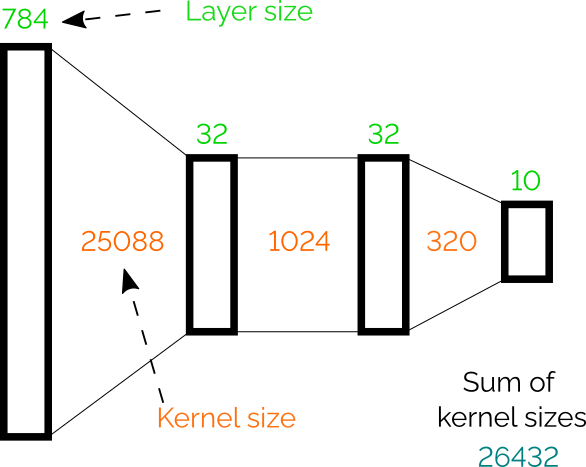This architecture gets 95+% accuracy on the MNIST test set after three epochs of training.

Pruning method. The lottery ticket hypothesis  claims that neural networks pruned, reset to their initial training weights, and retrained, and that this retrained network will often learn faster, attain better asymptotic performance and generalise better than the original network. The pruning method used in this paper is relatively simple:

• Initialize the neural network, and save the initialisation.

• Train the network to convergence.

• Prune X% of smallest weights per layer.

• Reset the network to its initialization,

• Repeat the above process, each time pruning a percentage of the weights.

Note that in our experiment we are pruning only only kernels, not biases. As there are only 74 weights in biases, this isn’t a consequential number of parameters compared to 27432, and it makes the implementation simpler.

Gaining insight. Having matrices with many zeros is not in itself helpful. We need to use an interpretation method to take advantage of this sparsity. Because we hope for quite a sparse representation at the end, chose to visualize the network as it’s flowgraph.

To demonstrate how this works, we’ll use the following computation:

This can be represented as the following flowgraph: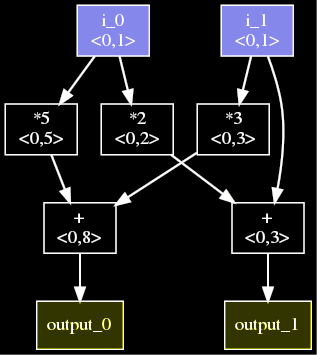• Each box produces a scalar value

• Blue nodes are inputs, and yellow nodes are outputs.

• <x,y> shows an a simple overapproximation of bounds. In this example we assume that the inputs are in the interval . Since the computation is simple, the calculated bounds for outputs are precise in this particular case.

# Initial results

When we apply the lottery ticket pruning method on all layers, we can prune the network to 2% of its original size (leaving only 539 weights) and still achieve 90.5% accuracy on test set. For more detail on the number of weights per layer see the following figure: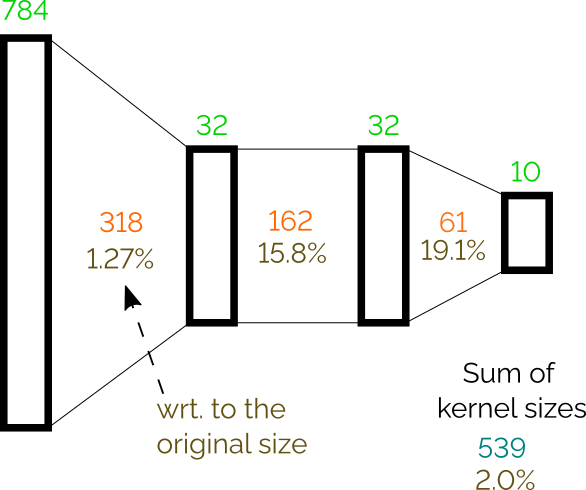Now we can apply our flow-graph visualisation on the pruned network. The output is a graph with 963 vertices and 1173 edges. Note that for this figure and all following, we are rounding values to two decimal places.

# Making it clearer

As we can see, the flowgraph is quite still quite dense, and it’s difficult to extract any insight into how the network behaves from it. To make it clearer, we can exploit the following observations: Many vertices and corresponding edges are from the input layer to the first hidden layer. We can detect dot product operations in the first layer and simply visualize weights as a 2D image, because the first layer is directly connected to the pixels. For example, instead of the following dot product:

(where is an input pixel at position ) we can instead use the following image: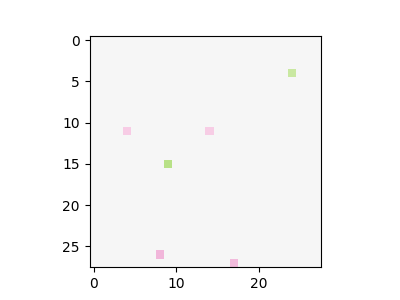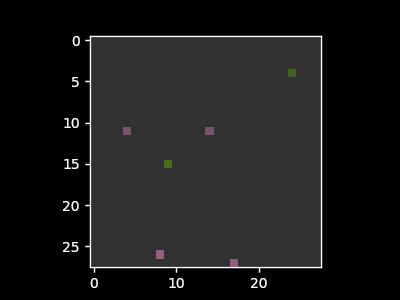Colour intensity is used to show absolute value; green is used for positive values; red for negative, and grey is the zero.

A further simplification is to display only subgraph of the flowgraph that is relevant for each output separately. While it loses information about what is reused between outputs, it provides more readable graphs.

Combining these two simplifications, we get the following visualisation:

Here we can observe that some filters on the input are relatively interpretable, and it is clear what shapes they detect: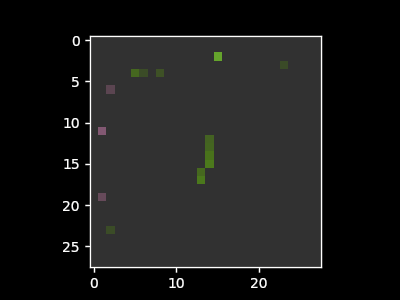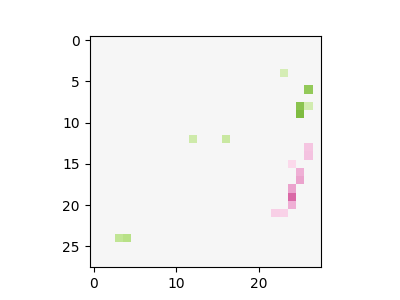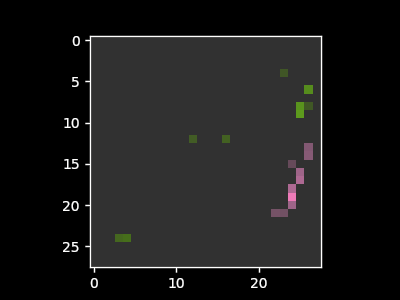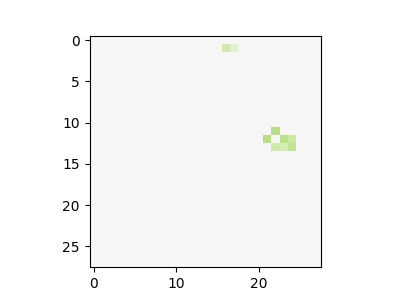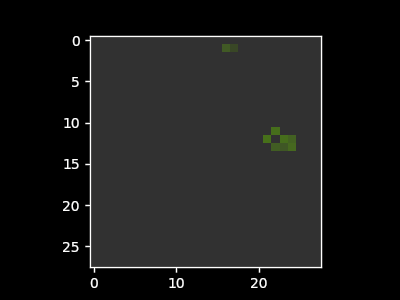On the other hand, some of them look more like random pixel detections: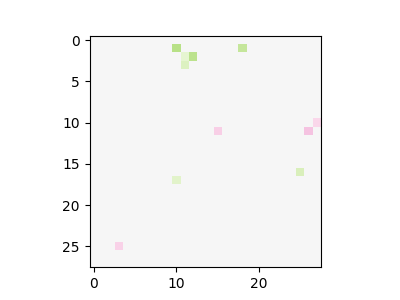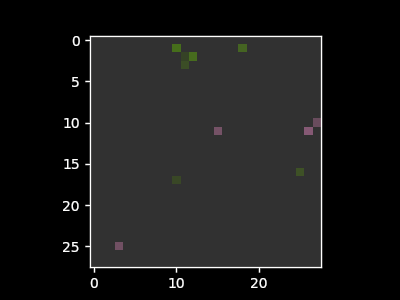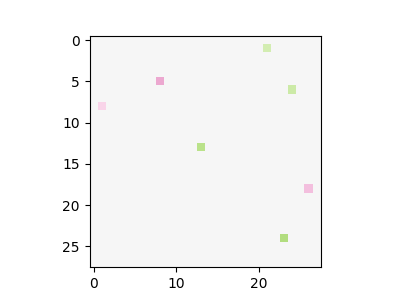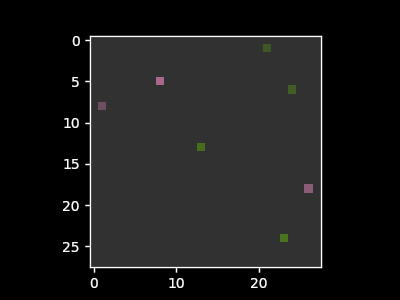The computation after the initial layer still remains relatively dense and hard to understand. It is now possible to track the flow of individual values through the graph in most places, but we can do better.

# Visualising behaviour on specific inputs

To get more insight, we can also visualize flowgraphs on individual inputs. This allows us to visualize values flowing between nodes; the thickness of lines corresponds to the absolute value of the scalar value produced by a source operation while green lines carry positive values, red lines carry negative values, and grey lines are exactly 0.

Also, for each visualized dot product, we show the result of applying this filter to the input image (that is, the result of the dot product multiplication of the input and the weights). This is shown as the right-hand figure next to the dot product filter visualisations at the top of the flowgraph.

The following figure shows this visualisation for six random images from the test set (the values in square brackets in the nodes are the actual values that flow through the graph):

The figure shows that it relatively trackable to see which part of the network contributes to the final decision. For example, let us see how the probability that the input is digit 0 (output_0) is computed. For the digit 1 input, it is clear that the decision to not classify it as a 0 is heavily influenced by one specific dot product that detects pixels in the middle of the figure. Another observation is that there are two main branches in the flow that plays an important role of classifying digit 0 correctly (i.e. digit 0 and output_0). It mixes together a detector of a bottom pixels and detection of some specific pixels. For the sixth input (digit 6) is used, it is not classified as 0 even though some of detectors are positively triggered (similarly to case with input 0); however, the detector of the central pixels overweights and produce a negative score.

# Pruning once more

Let us revisit the idea of pruning. We have pruned the network as much as possible to get a more understandable object then a fully connected network. However, there is space for trade-offs; we don’t need to prune each layer equally, and could trade off pruning some layers more to prune other layers less.

Specifically, in our case we have a visualization of the first layer who’s interpretability is not influenced as much by the sparsity. This allows us to completely disable pruning of the first layer. With this approach we get the following network flowgraph that has 93.5% accuracy on the test set: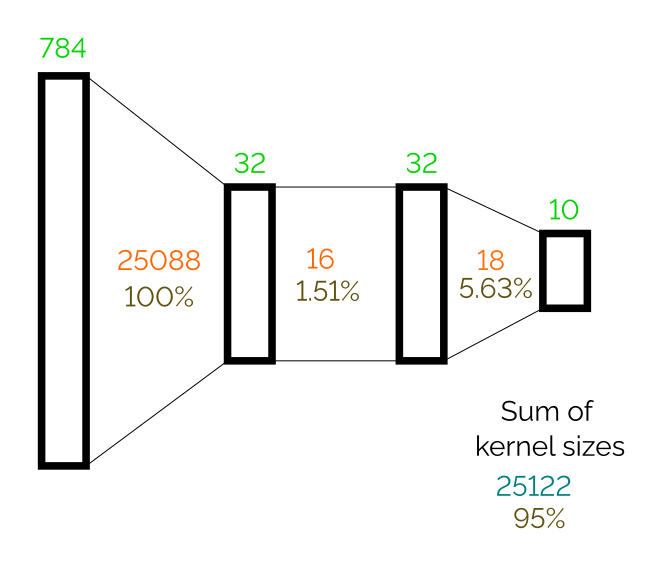The network has 95% of its original weights, but last two kernels are several times smaller than in the previous case. This gives us a significantly simpler flowgraph. It can be now easily shown in one diagram for each outputs without loosing clarity. The following figure shows resulting flow graphs applied on six figures from the testing test.

One interesting observation is that this network returns a constant value for output_2. This means that the digit 2 is a kind of reference point; an input is classified as digit 2 if other outputs are sufficiently suppressed.

We can again easily track down properties like digit 1 is not classified as 0 because of the pixel in the middle part. In this version, it is less clear what each dot products detects, but on the other hand it very straightforward what is happening with each dot product value in the rest of the computation.

# Domains other than MNIST

The approach of pruning and visualization of flow graphs may be also applied to other domains such as reinforcement learning for continuous control. We use the classic OpenAI CartPole-v0 environment. The following figure shows a network trained with DQN, and then pruned when reaching maximal score, on the CartPole environment. For this network, we can observe that one input (i_1, which is the cart velocity) is completely ignored, while the agent still gains the maximal score.

On the other hand, our approach doesn’t seem useful for convolutional neural networks, since applying the convolutional filters over the whole output of the previous layer is a part of the algorithm and it is not pruned with weights. Therefore, a convolutional filter even with a single weight may produce many connections in the flow graph. The presented approach still produces dense flow graphs even for heavily pruned convolutional networks.

The following figure shows a network created as 3 convolutional layers (6 channels, 3x3 filters, without padding, kernel sizes: 54, 324, 324) followed by a dense layer (kernel size: 29040, no activation). The layers was pruned to 6 (11%), 23 (7%), 23 (7%), and 135 (0.46%) of their original sizes. The resulting network has only 80% accuracy and quite a low number of weights, but still produces a relatively large and dense graphs. The graph for computing output_7 is the largest one (1659 vertices).

# Conclusion

While we may not have conclusively answered the question posed in the title, we have generated insight and intuitions with this simple example. As expected, pruning dense object may lead to more interpretable objects; however, there are trade-offs. Only counting the number of weights in the final object is probably not the ideal metric to get a most interpretable result. In our experiment, the later flowgraphs (of dense nets) where more interpretable despite having many more weights than the previous examples. This points to an answer to the opposing views of sparsity possibly giving both more and less interpretability; In some settings sparsity is beneficial in giving humans understanding and transparency, but when a visualisation which doesn’t rely on sparsity can still produce results that humans can understand and interpret, the sparsity isn’t necessarily useful.

Acknowledgement: This text was created as a part of AISRP.

Adversarial Robustness as a Prior for Learned Representations [PDF] Engstrom, L., Ilyas, A., Santurkar, S., Tsipras, D., Tran, B. and Madry, A., 2019.

The Lottery Ticket Hypothesis: Training Pruned Neural Networks  [PDF] Frankle, J. and Carbin, M., 2018. CoRR, Vol abs/​1803.03635.

• I feel like this is trying to apply a neural network where the problem specification says “please train a decision tree.” Even when you are fine with part of the NN not being sparse, it seems like you’re just using the gradient descent training as an elaborate img2vec method.

Maybe the idea is that you think a decision tree is too restrictive, and you want to allow more weightings and nonlinearities? Still, it seems like if you can specify from the top down what operations are “interpretable,” this will give you some tree-like structure that can be trained in a specialized way.

• Planned summary for the Alignment Newsletter:

If you want to visualize exactly what a neural network is doing, one approach is to visualize the entire computation graph of multiplies, additions, and nonlinearities. While this is extremely complex even on MNIST, we can make it much simpler by making the networks _sparse_, since any zero weights can be removed from the computation graph. Previous work has shown that we can remove well over 95% of weights from a model without degrading accuracy too much, so the authors do this to make the computation graph easier to understand.
They use this to visualize an MLP model for classifying MNIST digits, and for a DQN agent trained to play Cartpole. In the MNIST case, the computation graph can be drastically simplified by visualizing the first layer of the net as a list of 2D images, where the kth activation is given by the dot product of the 2D image with the input image. This deals with the vast majority of the weights in the neural net.

Planned opinion:

This method has the nice property that it visualizes exactly what the neural net is doing—it isn’t “rationalizing” an explanation, or eliding potentially important details. It is possible to gain interesting insights about the model: for example, the logit for digit 2 is always −2.39, implying that everything else is computed relative to −2.39. Looking at the images for digit 7, it seems like the model strongly believes that sevens must have the top few rows of pixels be blank, which I found a bit surprising. (I chose to look at the digit 7 somewhat arbitrarily.)
Of course, since the technique doesn’t throw away any information about the model, it becomes very complicated very quickly, and wouldn’t scale to larger models.
• I like this visualization tool. There are some very interesting things going on here when you look into the details of the network in the second-to-last MNIST figure. One is that it seems to mostly identify each digit by ruling out the others. For instance, the first two dot-product boxes (on the lower left) could be described as “not-a-0” detectors, and will give a positive result if they detect pixels in the center, near the corners, or at the extreme edges. The next two boxes could be loosely called “not-a-9“ detectors (though they also contribute to the 0 and 4 classifications) and the three after that are “not-a-4” detectors. (The use of ReLU makes this functionally different from having a “4” detector with a negative weight.)

Now, take a look at the two boxes that contribute to output 1 (I would call these “not-a-1” detectors). If you select the “7″ input and see how those two boxes respond to it, they both react pretty strongly to the very bottom of the 7 (the fact that it’s near the edge) and that’s what allows the network to distinguish a 7 from a 1. Intuitively, this seems like a shared feature—so why is the network so sure that anything near the bottom cannot be part of a 1?

It looks to me like it’s taking advantage of the way the MNIST images are preprocessed, with each digit’s center-of-mass translated to the center of the image. Because the 7 has more of its mass near the top, its lower extremity can reach farther from the center. The 1, on the other hand, is not top-heavy or bottom-heavy, so it won’t be translated by any significant amount in preprocessing and its extremities can’t get near the edges.

The same thing happens with the “not-a-3” detector box when you select input 2. The “not-a-3″ detector triggers quite strongly because of the tail that stretches out to the right. That area could never be occupied by a 3, because the 3 has most of its pixel mass near its right edge and will be translated left to get its center of mass centered in the image. The “7” detector (an exception to the pattern of ruling digits out) mostly identifies a 7 by the fact that it does not have any pixels near the top of the image (and to a lesser extent, does not have pixels in the lower-right corner).

What does this pattern tell us? First, that a different preprocessing technique (centering a bounding box in the image, for instance, instead of the digit’s center of mass) would require a very different strategy. I don’t know off hand what it would look like—maybe there’s some trick for making the problems equivalent, maybe not. Second, that it can succeed without noticing most of what humans would consider the key features of these digits. For the most part it doesn’t need to know the difference between straight lines and curved lines, or whether the parts connect the way they’re supposed to, or even whether lines are horizontal or vertical. It can use simple cues like how far each digit extends in different directions from its center of mass. Maybe with so few layers (and no convolution) it has to use those simple cues.

As far as interpretability, this seems difficult to generalize to non-visual data, since humans won’t intuitively grasp what’s going on as easily. But it certainly seems worthwhile to explore ideas for how it could work.